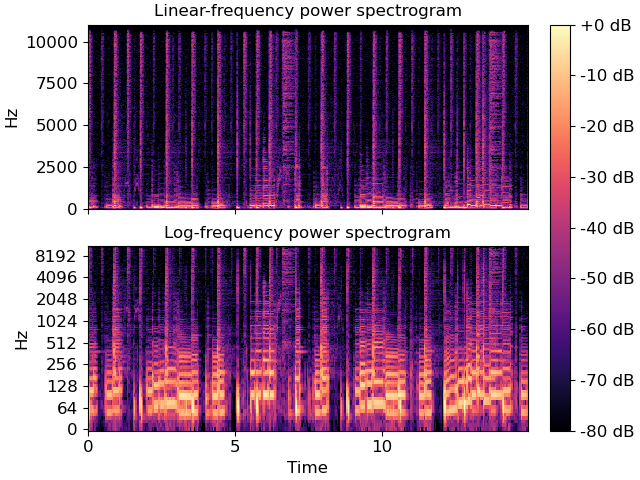# librosa.display.specshow¶

librosa.display.specshow(data, x_coords=None, y_coords=None, x_axis=None, y_axis=None, sr=22050, hop_length=512, fmin=None, fmax=None, tuning=0.0, bins_per_octave=12, key='C:maj', Sa=None, mela=None, thaat=None, auto_aspect=True, htk=False, ax=None, **kwargs)[source]

Display a spectrogram/chromagram/cqt/etc.

For a detailed overview of this function, see Using display.specshow

Parameters
datanp.ndarray [shape=(d, n)]

Matrix to display (e.g., spectrogram)

srnumber > 0 [scalar]

Sample rate used to determine time scale in x-axis.

hop_lengthint > 0 [scalar]

Hop length, also used to determine time scale in x-axis

x_axis, y_axisNone or str

Range for the x- and y-axes.

Valid types are:

• None, ‘none’, or ‘off’ : no axis decoration is displayed.

Frequency types:

• ‘linear’, ‘fft’, ‘hz’ : frequency range is determined by the FFT window and sampling rate.

• ‘log’ : the spectrum is displayed on a log scale.

• ‘fft_note’: the spectrum is displayed on a log scale with pitches marked.

• ‘fft_svara’: the spectrum is displayed on a log scale with svara marked.

• ‘mel’ : frequencies are determined by the mel scale.

• ‘cqt_hz’ : frequencies are determined by the CQT scale.

• ‘cqt_note’ : pitches are determined by the CQT scale.

• ‘cqt_svara’ : like cqt_note but using Hindustani or Carnatic svara

All frequency types are plotted in units of Hz.

Any spectrogram parameters (hop_length, sr, bins_per_octave, etc.) used to generate the input data should also be provided when calling `specshow`.

Categorical types:

• ‘chroma’ : pitches are determined by the chroma filters. Pitch classes are arranged at integer locations (0-11) according to a given key.

• chroma_h, chroma_c: pitches are determined by chroma filters, and labeled as svara in the Hindustani (chroma_h) or Carnatic (chroma_c) according to a given thaat (Hindustani) or melakarta raga (Carnatic).

• ‘tonnetz’ : axes are labeled by Tonnetz dimensions (0-5)

• ‘frames’ : markers are shown as frame counts.

Time types:

• ‘time’markers are shown as milliseconds, seconds, minutes, or hours.

Values are plotted in units of seconds.

• ‘s’ : markers are shown as seconds.

• ‘ms’ : markers are shown as milliseconds.

• ‘lag’ : like time, but past the halfway point counts as negative values.

• ‘lag_s’ : same as lag, but in seconds.

• ‘lag_ms’ : same as lag, but in milliseconds.

Rhythm:

• ‘tempo’markers are shown as beats-per-minute (BPM)

using a logarithmic scale. This is useful for visualizing the outputs of feature.tempogram.

• ‘fourier_tempo’same as ‘tempo’, but used when

tempograms are calculated in the Frequency domain using feature.fourier_tempogram.

x_coords, y_coordsnp.ndarray [shape=data.shape[0 or 1]+1]

Optional positioning coordinates of the input data. These can be use to explicitly set the location of each element `data[i, j]`, e.g., for displaying beat-synchronous features in natural time coordinates.

If not provided, they are inferred from `x_axis` and `y_axis`.

fminfloat > 0 [scalar] or None

Frequency of the lowest spectrogram bin. Used for Mel and CQT scales.

If `y_axis` is cqt_hz or cqt_note and `fmin` is not given, it is set by default to `note_to_hz('C1')`.

fmaxfloat > 0 [scalar] or None

Used for setting the Mel frequency scales

tuningfloat

Tuning deviation from A440, in fractions of a bin.

This is used for CQT frequency scales, so that `fmin` is adjusted to `fmin * 2**(tuning / bins_per_octave)`.

bins_per_octaveint > 0 [scalar]

Number of bins per octave. Used for CQT frequency scale.

keystr

The reference key to use when using note axes (cqt_note, chroma).

Safloat or int

If using Hindustani or Carnatic svara axis decorations, specify Sa.

For cqt_svara, `Sa` should be specified as a frequency in Hz.

For chroma_c or chroma_h, `Sa` should correspond to the position of Sa within the chromagram. If not provided, Sa will default to 0 (equivalent to C)

melastr or int, optional

If using chroma_c or cqt_svara display mode, specify the melakarta raga.

thaatstr, optional

If using chroma_h display mode, specify the parent thaat.

auto_aspectbool

Axes will have ‘equal’ aspect if the horizontal and vertical dimensions cover the same extent and their types match.

To override, set to False.

htkbool

If plotting on a mel frequency axis, specify which version of the mel scale to use.

• False: use Slaney formula (default)

• True: use HTK formula

See `core.mel_frequencies` for more information.

axmatplotlib.axes.Axes or None

Axes to plot on instead of the default plt.gca().

Arguments passed through to `matplotlib.pyplot.pcolormesh`.

By default, the following options are set:

• `rasterized=True`

• `shading='flat'`

• `edgecolors='None'`

Returns
colormesh`matplotlib.collections.QuadMesh`

The color mesh object produced by `matplotlib.pyplot.pcolormesh`

`cmap`

Automatic colormap detection

`matplotlib.pyplot.pcolormesh`

Examples

Visualize an STFT power spectrum using default parameters

```>>> import matplotlib.pyplot as plt
>>> y, sr = librosa.load(librosa.ex('choice'), duration=15)
>>> fig, ax = plt.subplots(nrows=2, ncols=1, sharex=True)
>>> D = librosa.amplitude_to_db(np.abs(librosa.stft(y)), ref=np.max)
>>> img = librosa.display.specshow(D, y_axis='linear', x_axis='time',
...                                sr=sr, ax=ax)
>>> ax.set(title='Linear-frequency power spectrogram')
>>> ax.label_outer()
```

Or on a logarithmic scale, and using a larger hop

```>>> hop_length = 1024
>>> D = librosa.amplitude_to_db(np.abs(librosa.stft(y, hop_length=hop_length)),
...                             ref=np.max)
>>> librosa.display.specshow(D, y_axis='log', sr=sr, hop_length=hop_length,
...                          x_axis='time', ax=ax)
>>> ax.set(title='Log-frequency power spectrogram')
>>> ax.label_outer()
>>> fig.colorbar(img, ax=ax, format="%+2.f dB")
```